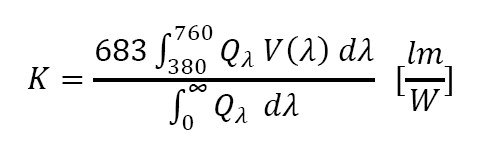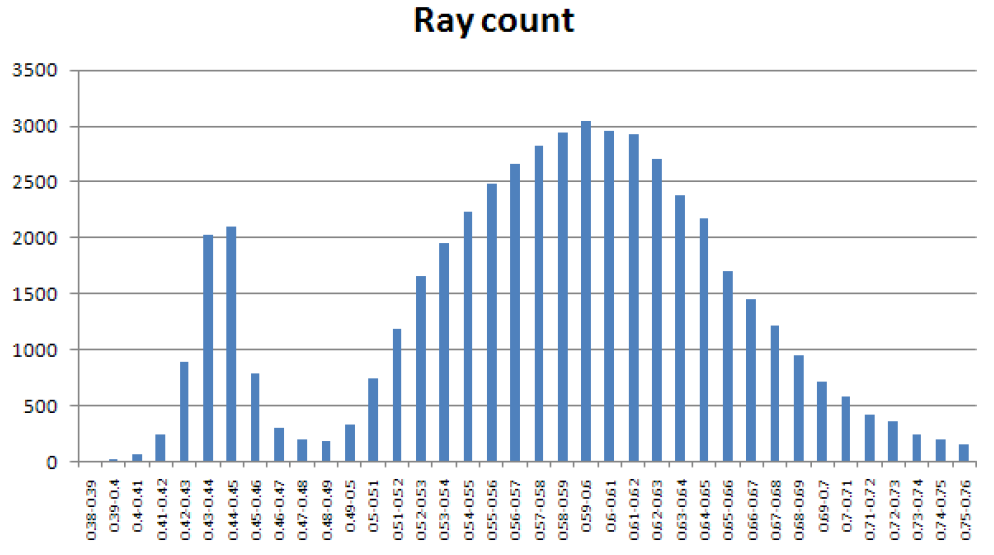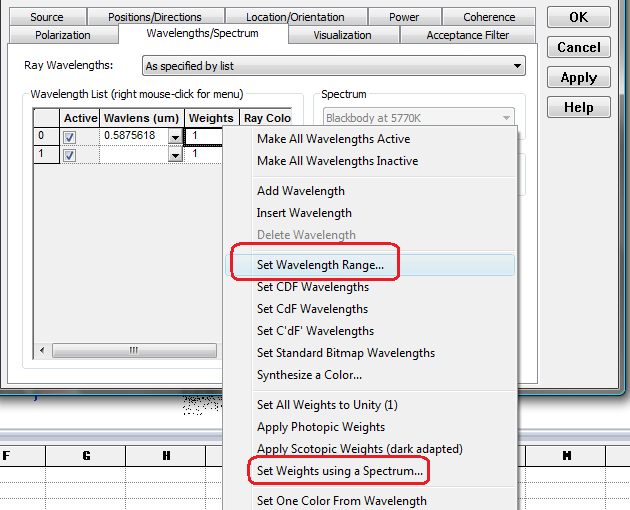FRED can express the power of its sources in either radiometric (Watts) or photometric (Lumens) units. This article discusses the methodology behind this feature.

The power of any light source can be expressed in either radiometric or photometric units. Radiometric units apply across the entire electro-magnetic spectrum and describe a wide variety of sources such as lasers, thermal radiation, microwaves, ultra-violet, etc. Photometric units are commonly used to characterize incandescent lamps, arc lamps, LEDs, etc., and apply to visible radiation only.

Some source types allow source power to be specified in either Watts or Lumens. If the Lumens option is selected, a spectrum from the Spectrum folder must be selected (either in the settings of the Source Primitive, or on the Wavelength Tab of the Detailed Source. FRED uses this spectrum to convert ray fluxes into radiometric units (W) before tracing. To determine the radiometric flux assigned to a photometric source, the power in lumens is divided by the luminous efficacy of the associated spectrum. The luminous efficacy K is defined as:where Q is the source spectrum and V is the photopic spectral luminous efficiency function.

Selection of a spectrum enables the option Randomly according to spectrum as shown in Figure 1. This option is a Monte-Carlo method where the spectrum assumes the role of a probability distribution in assigning wavelengths to individual rays. All rays have the same flux. The probability distribution will then dictate that more rays are created within a given wavelength range where the probability is high.

The user should be aware that Monte-Carlo wavelength generation introduces an additional element of chromatic noise to the inherent spatial noise arising from Poisson statistics commonly associated with raytracing. As a result, the ray count for such a source must be increased to take this fact into account.A second option is to use wavelengths specified in the Wavelength List. In this case, the user is free to select the number of discrete wavelengths over the desired range. Right-click in the Wavelength list table to access FRED’s Wavelength Range dialog as indicated by the red arrow in Figure 3. Once the list is set, right-click a second time to apply wavelength weights based upon a spectrum (blue arrow). The Spectrum selection in the right portion of the dialog is ignored when the As specified by list option is used. This configuration of the Wavelength Tab creates the same number of rays for each specified wavelength. The number of wavelengths selected will affect the accuracy of any color calculation.As a compliment to the photometric source type, FRED offers photometric Analysis functions such as Illuminance and Luminous Intensity (see Figure 4). Illuminance, measured in units of Lux (Lumens/m2), Phot (Lumens/cm2) or Footcandle (Lumens/ft2), is the photometric equivalent of the radiometric quantity Irradiance (Watts/area). Irradiance and Illuminance calculations use the conventional, planar Analysis Surfaces. Luminous Intensity, measured in units of candela (Lumens/sr) is the photometric equivalent to radiometric quantity Intensity (Watts/sr). Intensity (on a Polar Grid) and Luminous Intensity calculations use the Directional Analysis Entity.